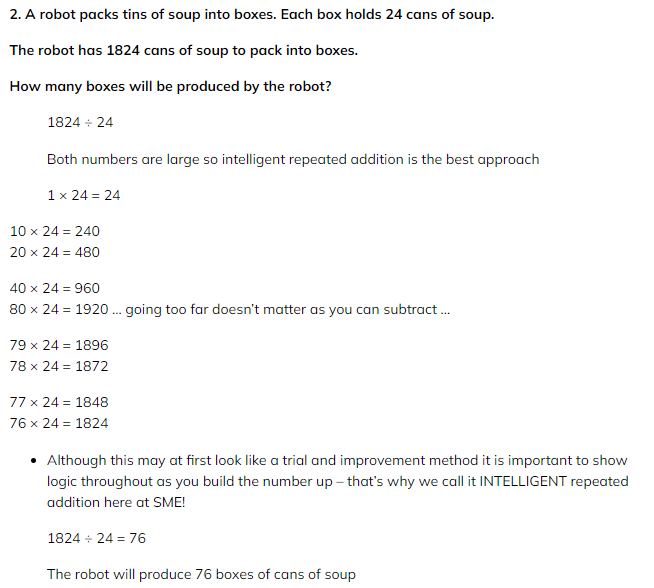# Edexcel IGCSE Maths 复习笔记 1.17.2 Division (non-Calc)

Edexcel IGCSE Maths 复习笔记 1.17.2 Division (non-Calc)

#### (Non-calculator) division – more methods

• Most students will have seen short division (bus stop method) and long division and there is often confusion between the two
• Fortunately, you only need one – so use short division
• While short division is best when dividing by a single digit, for bigger numbers you need a different approach
• You can use other areas of maths that you know to help – eg. cancelling fractions, “shortcuts” for dividing by 2 and 10, and the repeated addition (“chunking”) method covered in (Non-Calculator) Multiplication

#### 1. Short division (bus stop method)

• Apart from where you can use shortcuts such as dividing by 2 or by 5, this method is best used when dividing by a single digit

eg. 534 ÷ 6So, 534 ÷ 6 = 89

#### 2. Factoring & cancelling

• This involves treating division as you would if you were asked to cancel fractions
• You can use the fact that with division, most non-calculator questions will have only number answers
• The only thing to be aware of is that this might not be the case if you’ve been asked to write a fraction as a mixed number (but if you are asked to do that it should be obvious from the question)

eg. 1008 ÷ 28

1008 ÷ 28 = 504 ÷ 14 = 252 ÷ 7 = 36

• You may have spotted the first two values (1008 and 28) are both divisible by 4 which is fine but if not, divide top and bottom by any number you can
• To do the last part (252 ÷ 7) you can use the short division method above

• This is virtually identical to the version for multiplication – the process stops when the number dividing into is reachedeg 1674 ÷ 27This is the same as saying ? × 27 = 1674So we can build up in “chunks” of 27 until we get to 16741 × 27 = 2710 × 27 = 270

20 × 27 = 54040 × 27 = 1080

60 × 27 = 1620 … by using the last two results added together.Now you are close we can add on 27 one at a time again.61 × 27 = 1647

62 × 27 = 1674So 1674 ÷ 27 = 62

#### 4. Dividing by 10, 100, 1000, … (Powers of 10)

• This is a case of moving digits (or decimal points) or knocking off zeroseg. 380 ÷ 10 = 38

45 ÷ 100 = 0.45

#### 5. Dividing by 2, 4, 8, 16, 32, … (Powers of 2)

• This time it is a matter of repeatedly halvingeg. 280 ÷ 8 = 140 ÷ 4= 70 ÷ 2 = 35

1504 ÷ 32 = 752 ÷ 16 = 376 ÷ 8 = 188 ÷ 4 = 94 ÷ 2 = 47

#### Exam Tip

On the non-calculator paper, division is very likely to have a whole number (exact) answer.  So if, when using the repeated addition method, you do not reach this figure then it is likely you’ve made an error in your calculations somewhere.

Worked ExampleWorked Example